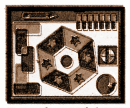# Samacheer Kalvi 8th Maths Book Solutions Term 1 Chapter 5 | Information Processing Answers For Tamil Nadu Board

Samacheer Kalvi 8th Maths Book Solutions Term 1 Chapter 5 – Information Processing is available here. The Samacheer Kalvi 8th Maths book answers of Term 1 Chapter 5, available at BYJU’S, contain step by step explanations designed by our Mathematics experts. All these important questions are based on the new pattern prescribed by the Tamil Nadu board. Students can also get the solutions of other chapters on Samacheer Kalvi 8th Maths solutions. Find here below, the compilation of Samacheer Kalvi 8th Maths Book Solutions Term 1 Chapter 5 Questions for the students to practice.

## Samacheer Kalvi Class 8 Maths Textbook Term 1 Chapter 5 Questions and Solutions

Term 1 Chapter 5 of the Samacheer Kalvi 8th Maths guide will help the students to solve problems related to the principles of counting, SET – game, map colouring, graph colouring.

### Samacheer Kalvi 8th Maths Term 1 Chapter 5: Information Processing Book Exercise 5.1 Questions and Solutions

Question 1: You are going to have an ice cream or a cake. There are three flavours (chocolate, strawberry, vanilla) in ice creams and two flavours (orange or red velvet) in the cake. In how many possible ways can you choose ice cream or the cake?

Solution:

We are going to either have ice cream or cake.

Ice cream can be selected from 3 flavours and cake from 2 flavours.

Both events cannot occur simultaneously.

Number of possible ways = 3 + 2 = 5 ways

Question 2: In how many ways, can the teacher choose 3 students in all, one each from 10 students in VI std, 15 students in VII std and 20 students in VIII std to go to an excursion?

Solution:

The teacher can select one student from class VI out of 10 students in 10 ways.

From class VII, out of 15 students in 15 ways and from class VIII, out of 20 students in 20 ways.

Number of ways of selection = 10 + 15 + 20 = 45 ways

Question 3: A safety locker in a jewel shop requires a 4 digit unique code. The code has the digits from 0 to 9. How many unique codes are possible?

Solution:

The unique code has 4 digits.

Each digit is formed using any of the 10 numbers from 0 to 9.

Hence, a single-digit number can be formed in 10 ways.

A double-digit number can be formed in 10 x 10 ways.

A three-digit number can be formed in 10 x 10 x 10 ways.

A four-digit number can be formed in 10 x 10 x 10 x 10 = 10,000 ways.

### Samacheer Kalvi 8th Maths Term 1 Chapter 5: Information Processing Book Exercise 5.2 Questions and Solutions

Question 1: Colour the following patterns with as few colours as possible but make sure that no two adjacent sections are of the same colour.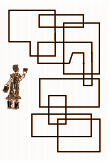Solution: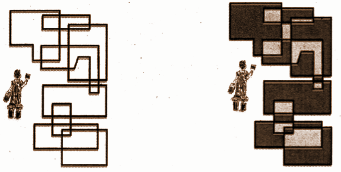Question 2: Ramya wants to paint a pattern in her living room wall with a minimum budget. Help her to colour the pattern with 2 colours but make sure that no two adjacent boxes are the same colour. The pattern is shown in the picture.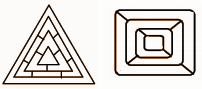Solution: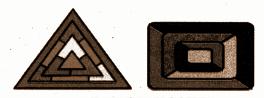Question 3: Colour the countries in the following maps with as few colours as possible but make sure that no two adjacent countries are of the same colour.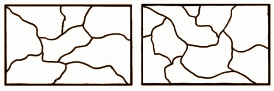Solution: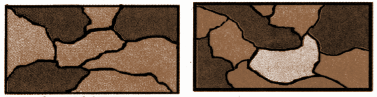### Samacheer Kalvi 8th Maths Term 1 Chapter 5: Information Processing Book Exercise 5.3 Questions and Solutions

Question 1: An examination paper has 3 sections, each with five questions and students are instructed to answer one question from each section. In how many different ways can the questions be answered?

Solution:

The tree diagram will be: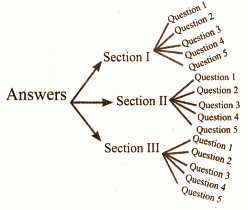Number of possible ways to select one question from each of 3 sections = 3 * 5 = 15 ways.

Question 2: On sports day, students must also take part in one of the one track events 100m Running and 4 × 100 m Relay. He must take part in any of the field events Long Jump, High Jump and Javelin Throw. In how many different ways can the student take part in the given events?

Solution:

Number of track events = (100m run, 4 * 100m relay) 2.

Number of field events = (Long jump, High jump, Javelin throw) 3.

The number of ways that students can take part in the given events = 2 * 3 = 6 ways.

Question 3: Colour the following pattern with as few colours as possible but make sure that no two adjacent sections are of the same colour.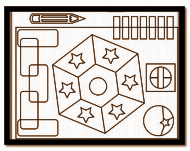Solution: